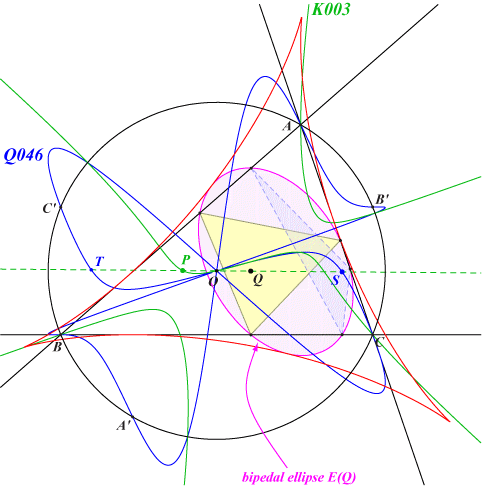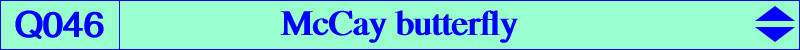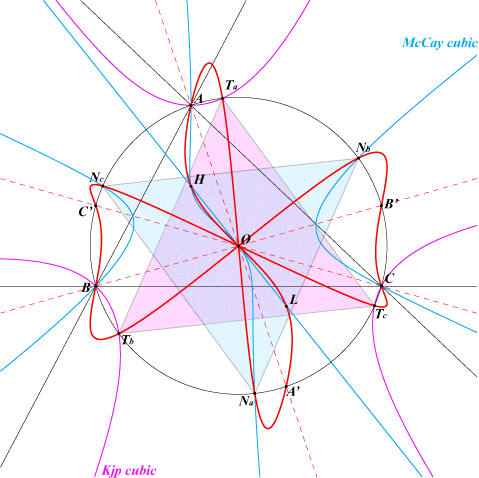too complicated to be written here. Click on the link to download a text file.X(3), X(4), X(20) Na, Nb, Nc : vertices of the circumnormal triangle Ta, Tb, Tc : vertices of the circumtangential triangle A', B', C' : vertices of the reflection triangle of ABC in O infinite points of the altitudes (singular points)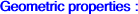The locus of point P such that the circumcevian triangle of P and ABC are orthologic is the McCay cubic. The locus of the orthology centers is the McCay cubic itself and Q046, the McCay butterfly. More precisely, if P is a point on the McCay cubic and if PaPbPc is the circumcevian triangle of P, the perpendiculars through A, B, C to the sidelines of PaPbPc concur at the isogonal conjugate P* of P. Thus, P* is one of the centers of orthology and obviously lies on the McCay cubic. The other center of orthology is Q on the line OPP* and on Q046. Q046 is a central sextic with center O which is a quadruple point on the curve. The four tangents at O pass through the in/excenters. The tangent at H passes through X(54), the Kosnita point. Q046 meets the line at infinity on the altitudes of ABC, these three points are isolated double (singular) points on the curve. If P and P* are two isogonal conjugates on the McCay cubic, the corresponding orthology centers Q, Q' are symmetric in O and these five points lie on a same line.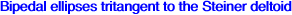It is known that the locus of the orthopole of a line pivoting around a fixed point Q is an ellipse E(Q) tritangent to the Steiner deltoid. Its center is the midpoint of HQ. When P ≠ O, its axes are parallel to the Simson lines of the intersections of the line OP with the circumcircle. E(O) is the nine point circle. E(Q) always contains the vertices of the (yellow) pedal triangle of Q and meets the sidelines of ABC again at three other points which are generally not the vertices of the pedal triangle of some point S unless the reflection P of Q about O lies on the McCay cubic K003. In this case, S lies on the McCay butterfly and so does its reflection T about O. E(Q) is then called a bipedal ellipse. T is the center of orthology (other than the isogonal conjugate of P) of ABC and the circumcevian triangle of P. Naturally, when Q lies on the Darboux cubic K004, the pedal triangle of Q is the cevian triangle of some point on the Lucas cubic K007 and E(Q) is a bicevian ellipse tritangent to the Steiner deltoid.